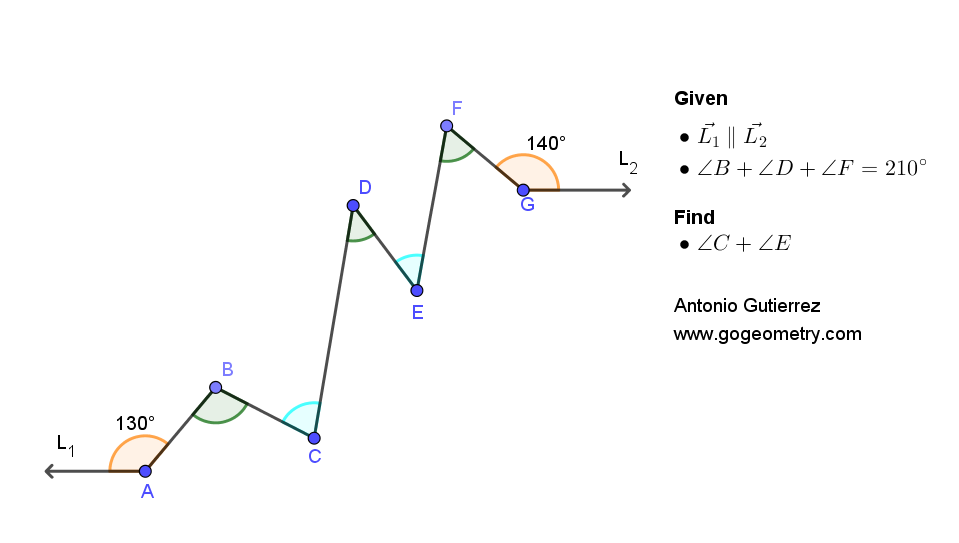# Geometry Problem 1506: Parallel Rays, Angles. Difficulty Level: High School.

Given the figure with parallel rays L1 and L2, with angle A measuring 130 degrees and angle G measuring 140 degrees, if the sum of the measures of angles B, D, and F is 210 degrees, find the sum of the measures of angles C and E## Definitions and Suggestions

• A ray is a straight line with a single endpoint that extends infinitely in one direction.
• An angle is a figure formed by two rays, or half-lines, that have a common endpoint and extend in different directions.
• Parallel lines are two lines in a plane that never meet or intersect, no matter how far they are extended.
• Suggestion:
Corresponding angles are angles that have the same relative position in each figure. When two lines are parallel, the corresponding angles are equal in measure.
If two lines are parallel, then the alternate interior angles formed by a transversal line cutting across the two lines are congruent.